Worksheet Solution: Can We Share

# Worksheet Solution: Can We Share - Notes | Study Mathematics for Class 3: NCERT - Class 3

 1 Crore+ students have signed up on EduRev. Have you?

Q.1. Fill in the blanks with the appropriate answer.
(i) 36 ÷ 6 = _____
Ans.
6

(ii) 45 ÷ 9 = _____
Ans.
5

(iii) 28 ÷ 4 = _____
Ans.
7

(iv) 88 ÷ 2 = _____
Ans.
44

(v) 63 ÷ _____ = 21
Ans.
3

(vi) 48 ÷ 8 = ____
Ans.
6

(vii) 56 ÷ 7 = _____
Ans.
8

(viii) 244 ÷ _____ = 122
Ans.
2

(ix) 99 ÷ 3 = _____
Ans.
33

(x) 72 ÷  _____ = 9
Ans.
8

(xi) 66 ÷ 2 = _____
Ans.
33

(xii) 55 ÷ _____ = 5
Ans.
11

(xiii) 81 ÷ _____ = 9
Ans.
9

(xiv) 90 ÷ 9 = _____
Ans.
10

(xv) 448 ÷ _____ = 112
Ans.
4

(xvi) _____ ÷ 5 = 7
Ans.
35

(xvii) 777 ÷ ____ = 7
Ans.
111

(xviii) 120 ÷ 6 = _____
Ans.
20

(xix) _____ ÷ 2 = 144
Ans.
882

(xx) 49 ÷ _____ = 7
Ans.
7

Q.2. I. Fill in the blanks:

 Multiplication fact Division fact 1 × 3 = ___ 3 ÷ 3 = ___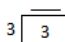2 × 3 = ___ 6 ÷ 3 = ___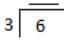3 × 3 = ___ 9 ÷ 3 = ___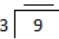4 × 3 = ___ 12 ÷ 3 = __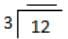5 × 3 = ___ 15 ÷ 3 = ___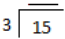6 × 3 = ___ 18 ÷ 3 = ___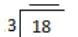7 × 3 = ___ 21 ÷ 3 = __ _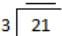8 × 3 = ___ 24 ÷ 3 = ___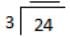9 × 3 = ___ 27 ÷ 3 = ___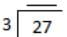10 × 3 = ___ 30 ÷ 3 = ___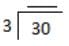Ans.

 3 1 1 6 2 2 9 3 3 12 4 4 15 5 5 18 6 6 21 7 7 24 8 8 27 9 9 30 10 10

II. Write two division facts for each multiplication fact:
(i) 6 × 3 = 18
Ans.
18 ÷ 3 = 6 and 18 ÷ 6 = 3

(ii) 2 × 3 = 6
Ans.
6 ÷ 2 = 3 and 6 ÷ 3 = 2

(iii) 9 × 3 = 27
Ans.
27 ÷ 9 = 3 and 27 ÷ 3 = 9

(iv) 12 × 3 = 36
Ans.
36 ÷ 3 = 12 and 36 ÷ 12 = 3

(v) 8 × 3 = 24
Ans.
24 ÷ 3 = 8 and 24 ÷ 8 = 3

III. Write two multiplication facts for each division fact:
(i) 15 ÷ 3 = 5
Ans.
5 × 3 = 15 and 3 × 5 = 15

(ii) 3 ÷ 3 = 1
Ans.
1 × 3 = 3 and 3 × 1 = 3

(iii) 21 ÷ 3 = 7
Ans.
7 × 3 = 21 and 3 × 7 = 21

(iv) 30 ÷ 3 = 10
Ans.
10 × 3 = 30 and 3 × 10 = 30

(v) 33 ÷ 3 = 11
Ans.
11 × 3 = 33 and 3 × 11 = 33

The document Worksheet Solution: Can We Share - Notes | Study Mathematics for Class 3: NCERT - Class 3 is a part of the Class 3 Course Mathematics for Class 3: NCERT.
All you need of Class 3 at this link: Class 3

## Mathematics for Class 3: NCERT

52 videos|84 docs|44 tests
 Use Code STAYHOME200 and get INR 200 additional OFF

## Mathematics for Class 3: NCERT

52 videos|84 docs|44 tests

Track your progress, build streaks, highlight & save important lessons and more!

,

,

,

,

,

,

,

,

,

,

,

,

,

,

,

,

,

,

,

,

,

;